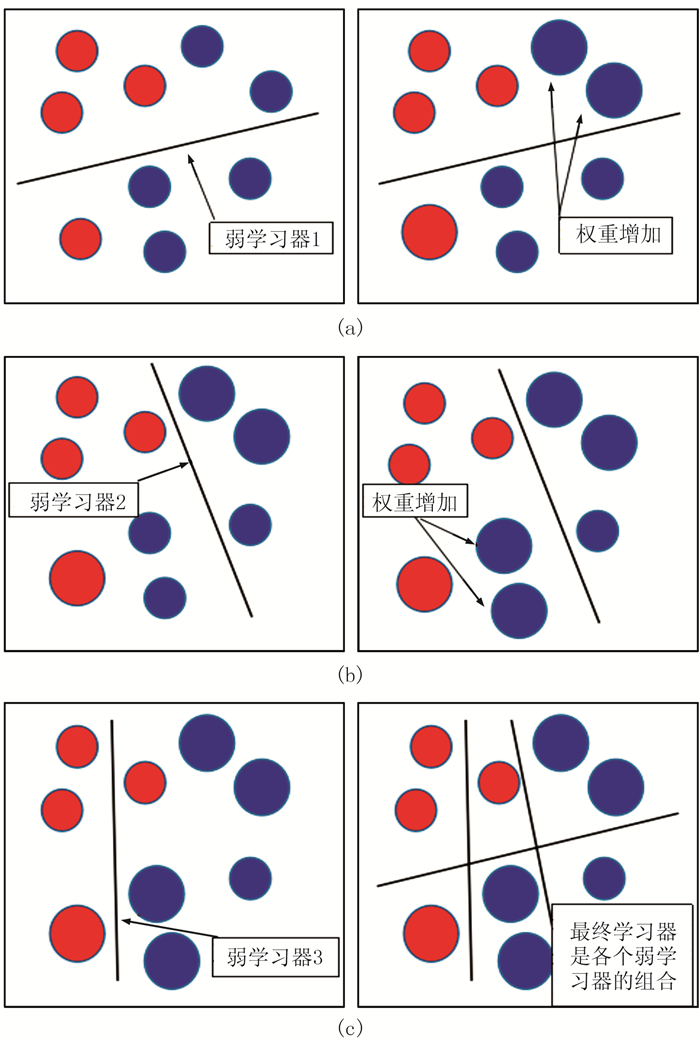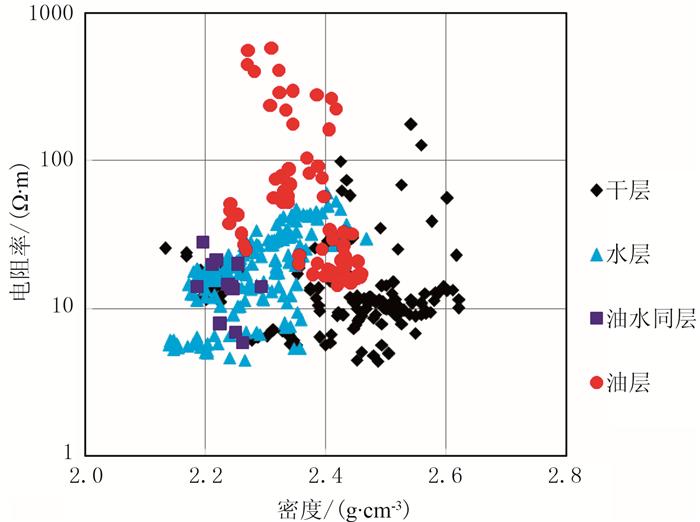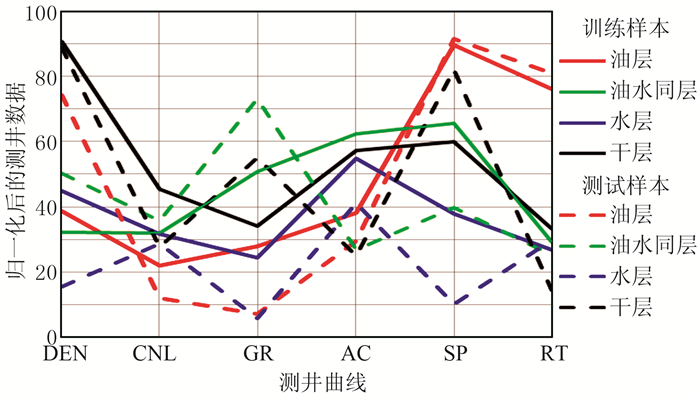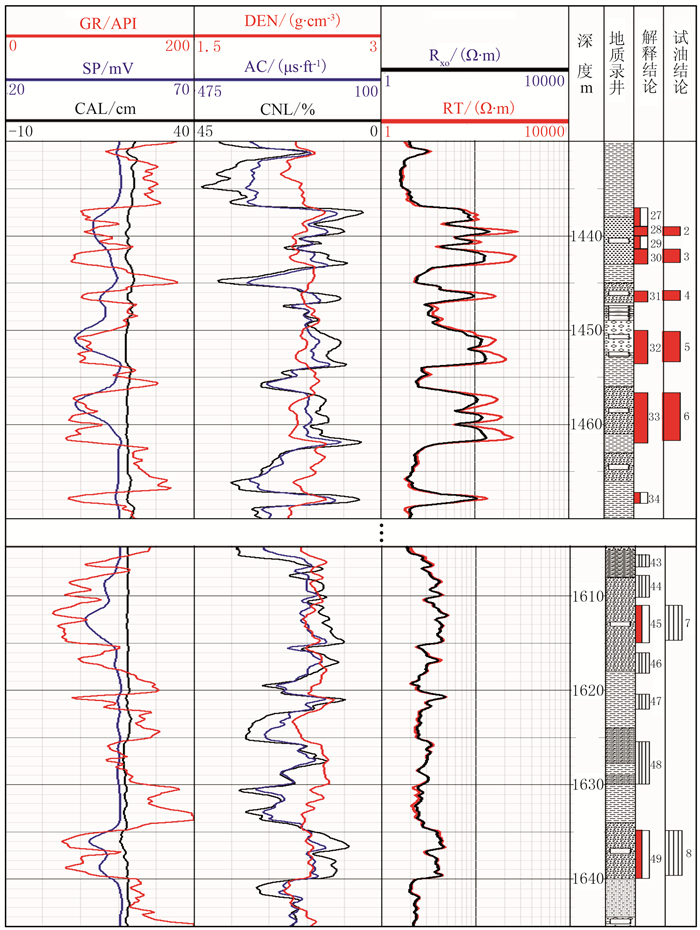石油地球物理勘探2019, Vol. 54Issue (6): 1357-1362  DOI: 10.13810/j.cnki.issn.1000-7210.2019.06.0200文章快速检索 高级检索

### 引用本文CHEN Ganghua, LIANG Shasha, WANG Jun, DI Shuhua, ZHUGE Yueying, LIU Youji. Fluid identification in glutenites with the machine learning AdaBoost.M2 algorithm. Oil Geophysical Prospecting, 2019, 54(6): 1357-1362. DOI: 10.13810/j.cnki.issn.1000-7210.2019.06.020.### 作者简介梁莎莎, 山东省青岛市黄岛区长江西路66号中国石油大学(华东)地球科学与技术学院, 266580。Email:1150374142@qq.com

### 文章历史

① 中国石油大学(华东)地球科学与技术学院, 山东青岛 266580;
② 嘉兴市佳安燃气技术服务有限公司, 浙江嘉兴 314000;
③ 中国石化胜利油田分公司勘探开发研究院, 山东东营 257015;
④ 中国石油集团测井有限公司华北分公司, 河北任丘 062550

Fluid identification in glutenites with the machine learning AdaBoost.M2 algorithm
CHEN Ganghua , LIANG Shasha①②, WANG Jun , DI Shuhua , ZHUGE Yueying , LIU Youji
① School of Geosciences, China University of Petroleum(East China), Qingdao, Shandong 266580, China;
② Jiaxing Jia'an Gas Technology Service Limited, Jiaxing, Zhejiang 314000, China;
③ Research Institute of Exploration and Development, Shengli Oilfield Branch Co., SINOPEC, Dongying, Shandong 257015, China;
④ North China Branch, Logging Co., Ltd., CNPC, Renqiu, Hebei 062550, China
Abstract: Glutenites are usually characterized by complex compositions, various pore structures, and strong heterogeneity, and the logging response of fluid is much smaller than that of gravel skeleton.So fluids in glutenites cannot be identified with conventional logging methods.Therefore we propose the machine learning AdaBoost.M2 algorithm for glutenite fluid identification.First combined with oil test data, the AdaBoost.M2 algorithm disassembles the K-class multi-fluid type classification problem into a two-class problem, and the sample distribution is obtained through multi-iterations.Then the decision tree algorithm called a weak learning algorithm obtains automatically a classifier ht for the discrimination.The proposed algorithm is applied to the reservoir fluid identification in A area.The sample return accuracy is 95% and the test accuracy is 91.5%, which proves the validity and applicability of proposed algorithm.
Keywords: machine learning    AdaBoost.M2 algorithm    glutenite    fluid identification
0 引言

1 方法原理图 1 AdaBoost算法原理示意图 左为弱学习器训练过程，右为训练结果

(1) 对于二分类问题，在初始化时对训练集中的每一个样本数据赋予同样的权重，并训练出一个弱学习器1(图 1a左)；

(2) 根据弱学习器1对样本数据的训练误差率更新样本数据的训练权重，使之前弱学习器1分类错误的训练样本点的权重增加，并使错误数据点被下一个弱学习器选中的概率增加(图 1a右)；

(3) 基于调整权重后的训练样本训练弱学习器2(图 1b左)；

(4) 重复上述(2)和(3)的步骤直至弱学习器数目达到事先指定的数目(图 1b右图 1c左)；

(5) 将各个训练得到的弱学习器组合得到最终的强分类器(图 1c右)。

AdaBoost.M2算法反复调用给定的弱学习器算法，主要是在训练集中维护一套权重分布。在第t轮迭代时，样本(xi, yi)(i为样本编号，xi为样本数据，yi为该样本的标签)的分布权值记为Dt(i)。初始所有样本权重相等，但进行迭代时，每一轮错误分类的样本权重都将会增加。弱学习器的任务就是根据分布Dt(i)找到合适的分类器。对于给定样本数据xi，有正确分类yi和非正确分类y(除yi之外的K-1类)。调用弱学习算法自动得到分类器ht，并假设ht从[0, 1]取值。对于样本(xi, yi)，ht会进行K-1次判别，每一次判别都有三种情况：当ht(xi, yi)=1、ht(xi, y)=0时，则分类正确，xi的类别是yi；当ht(xi, yi)=0、ht(xi, y)=1时，则分类错误，xi的类别是y；当ht(xi, yi)=ht(xi, y)时，则xi的类别随机从yiy中选取一个。

 $\frac{1}{2}\left[1-h_{t}\left(x_{i}, y_{i}\right)+h_{t}\left(x_{i}, y\right)\right]$ (1)

 $\frac{1}{K-1} \sum\limits_{y \neq y_{i}} \frac{1}{2}\left[1-h_{t}\left(x_{i}, y_{i}\right)+h_{t}\left(x_{i}, y\right)\right]\\ \;\;\;=\frac{1}{2}\left[1-h_{t}\left(x_{i}, y_{i}\right)+\frac{1}{K-1} \sum\limits_{y \neq y_{i}} h_{t}\left(x_{i}, y\right)\right]$ (2)

 $\frac{1}{2}\left[1-h_{t}\left(x_{i}, y_{i}\right)+\sum\limits_{y \neq y_{i}} q_{t}(i, y) h_{t}\left(x_{i}, y\right)\right]$ (3)

 \begin{aligned} \varepsilon_{t}=& \frac{1}{2} \sum\limits_{i=1}^{N} D_{t(i)}\left[1-h_{t}\left(x_{i}, y_{i}\right)+\right.\\ &\left.\sum\limits_{y \neq y_{i}} q_{t}(i, y) h_{t}\left(x_{i}, y\right)\right] \end{aligned} (4)

(1) 输入样本总数为N的训练集S={(x1, y1), …, (xi, yi), …, (xN, yN)}，标签yiY={1, 2, K}，其中i为样本编号，K是类别数。

(2) 初始化。样本数据初始分布$D_{1}(i)=\frac{1}{N}$，首次迭代中样本i的某个错误标签y的权重

 $\omega_{i, y}^{i}=\frac{D_{1}(i)}{K-1} \quad i=1, \cdots, N, y \in \boldsymbol{Y}=\left|\left\{y_{i}\right\}\right|$ (5)

(3) 循环迭代t=1, …, T

① 第t次迭代中样本i的的错误标签的权重之和$W_{i}^{t}=\sum\limits_{y \neq y_{i}} \omega_{i, y}^{t}$。对yyi$q_{t}(i, y)=\frac{\omega_{i, y}^{t}}{W_{i}^{t}}$，样本分布$D_{i}^{t}=\frac{W_{i}^{t}}{\sum\limits_{i=1}^{N} W_{i}^{t}}$

② 调用弱学习算法，传递Ditqt(i, y)给它，返回假设ht:X×Y→[0, 1]。X×Y为类别权重函数。

③ 计算ht的伪损失。

④ 置权重更新系数$\beta_{t}=\frac{\varepsilon_{t}}{1-\varepsilon_{t}}$

⑤ 计算新的权重

 $\omega_{i, y}^{i+1}=\omega_{i, y}^{i} \cdot \beta_{t}^{\frac{1}{2}\left[1+h_{t}\left(x_{i}, y_{i}\right)-h_{t}\left(x_{i}, y\right)\right]}$ (6)

(4) 输出T次循环后得到的最终组合分类器

 $h_{f(x)}=\arg \max \limits_{y \in {\boldsymbol{Y}}} \sum\limits_{t=1}^{T} \lg \frac{1}{\beta_{t}} h_{t}(x, y)$ (7)
2 应用实例

A研究区砂砾岩具有近物源、快速堆积、纵向厚度变化大、相变快等特点。砾石成分复杂、孔隙结构多样、非均质性强，存在多油水系统，油水层测井响应特征差异不明显。通常自然电位(SP)异常幅度可以反映储层渗透性、地层水矿化度，电阻率(RT)大小取决于孔隙结构以及孔隙所含流体。但在复杂砂砾岩储层中，地层水矿化度差异大，砂砾岩体岩石骨架对电阻率的的影响远远大于流体，利用常规测井资料难以准确评价油、水层。图 2 研究区电阻率—密度交会图图 3 归一化后的参数平行坐标系表 1 测试结果与试油、试采结果对照表图 4 X井试油段解释成果图 Rxo为冲洗带电阻率
3 结束语